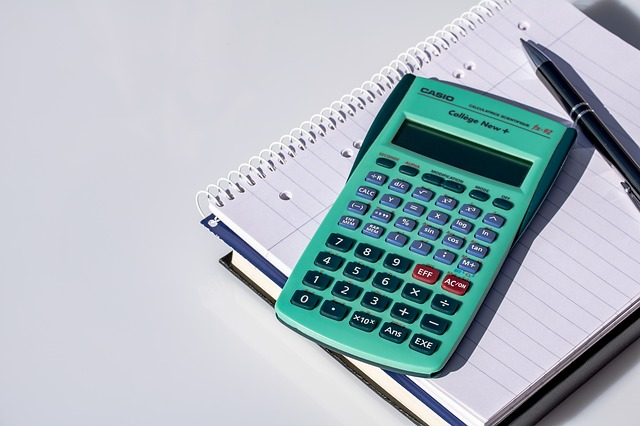# ElasticityElasticity is one of the most important terms in economics, and has a plethora of uses.  Economists define elasticity as the ratio of the percent change in one variable to the percent change in another valuable.

Its purpose is to measure how one variable responds to changes in another variable.

As an example, consider a company that sells 100 units of a product for \$20 each. Elasticity is used to explain what will happen to sales, if the price of the product is increased to \$21 a unit.

An “Elastic” variable is when a variable (sales of the newly priced unit) responds “a lot” to small changes in the other parameter (price.)

Contrary, an “inelastic” variable is when a variable (again, sales) responds “very little” to changes in the other parameter (price).

Price Elasticity of demand

Price elasticity of demand measures the change in percentage of demand caused by a percent change in price.

If the elasticity is between 0-1, demand is said to be inelastic (little change).

Greater than 1, demand is said to be elastic (great change).

As a note, it is common that the formula will yield a negative value, thus we concern ourselves with its positive value (i.e absolute value).

Elasticity (Ed) can be calculated as follows:

% change in quantity demanded
Ed  = __________________________
% change in price

Example: A company has conducted a survey that indicates consumer responses to a hypothetical increase in their price.  The company has discovered that if the price is increased from \$100 to \$106 (representing a 6% change), the quantity demanded will decrease by 8% (from 100 units sold to 92 units.)

Ed = (-8% / 6%) = -1.33

Since 1.33 is greater than 1, we can conclude that the demand is elastic, meaning that the change in demand caused by the change in price is considered “a lot.”

We can also calculate the effect of the change on price on revenue to get another view of what the Elasticity of demand means:

Old price, total revenue = 100 units sold at \$100 = \$10,000

New price, total revenue = 92 units sold at \$106 = \$9,752

Income Elasticity of Demand

Income elasticity of demand (IEOd) measures the response of a given good to a change in the income of its existing clients.  It is calculated as follows:

% change in quantity demanded
IEOd  =  _____________________
% change in real income

Example:  A company has conducted marketing surveys and discovered that if a consumer group’s income increases by 15%, the demand for the given good increases by 25%.
25%
IEOd = _____   = 1.66
15%

A negative Income Elasticity of Demand is generally associated with “inferior goods.”  The increase in income results in a decrease in demand for these products that are deemed to be of an inferior quality.  Examples of such products may be bus services, hamburger meat or generic brand soda.  When income rises, the consumer may opt to purchase an automobile, steak, and brand name cola, since they can now afford these extra luxuries.

A positive Income Elasticity of Demand is associated with “normal goods.”  An increase in income will lead to an increase in demand, for example tobacco has been calculated to have an elasticity of demand of 0.64.  An elasticity of greater than 1 is considered a “luxury good” for example a Mercedes Benz automobile or an Armani suit

A zero income elasticity occurs when an increase in income does not associate with a change in the demand for a good.  For example these are products that are essential to live, such as fresh vegetables and milk.

Conclusion

Elasticity is a widely used economic theory that has an extremely large range of uses and applications.  Other examples of how elasticity is used include its effect on international trade, analysis of advertising on consumer demand and analysis of future consumption patters.